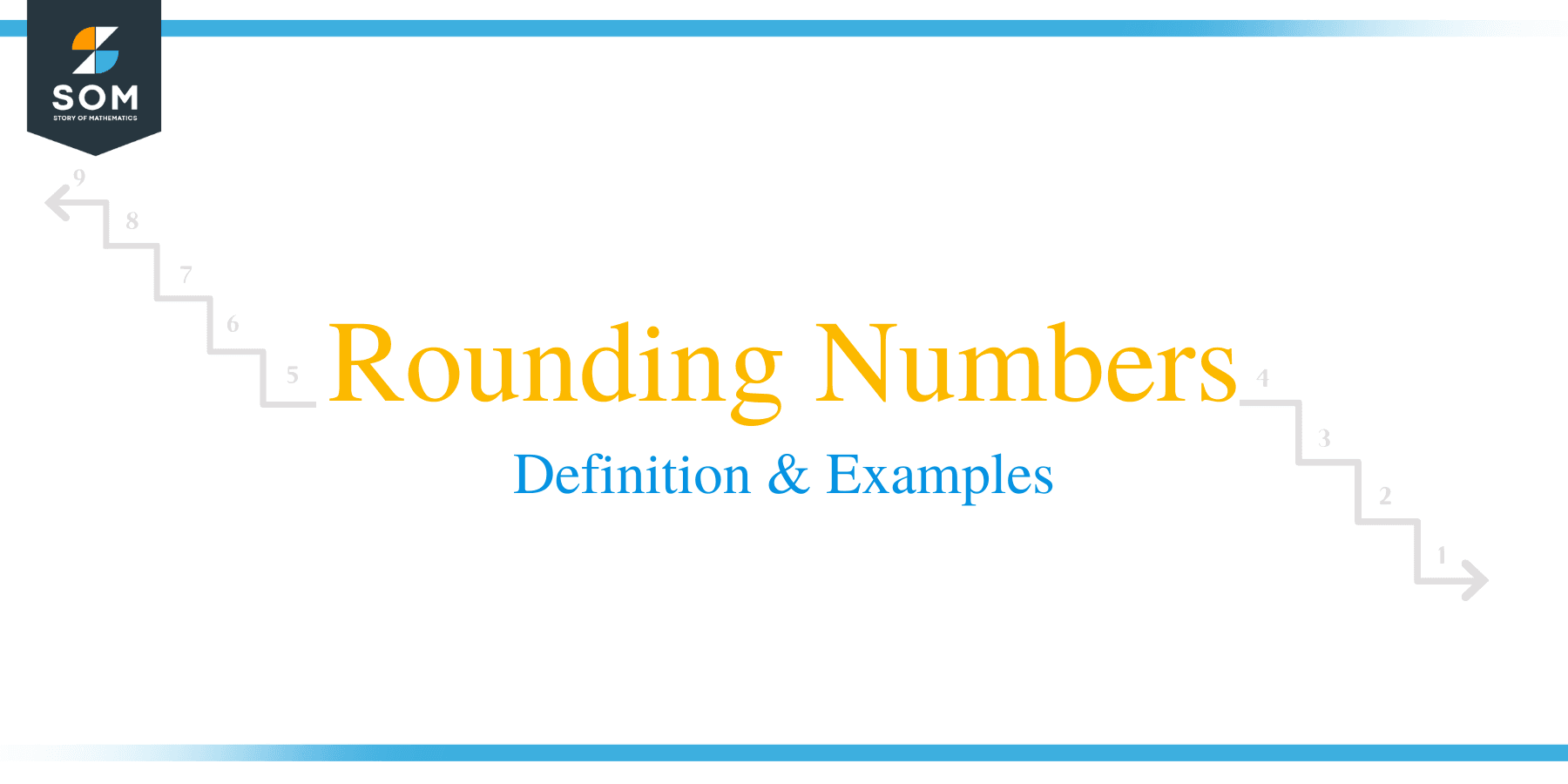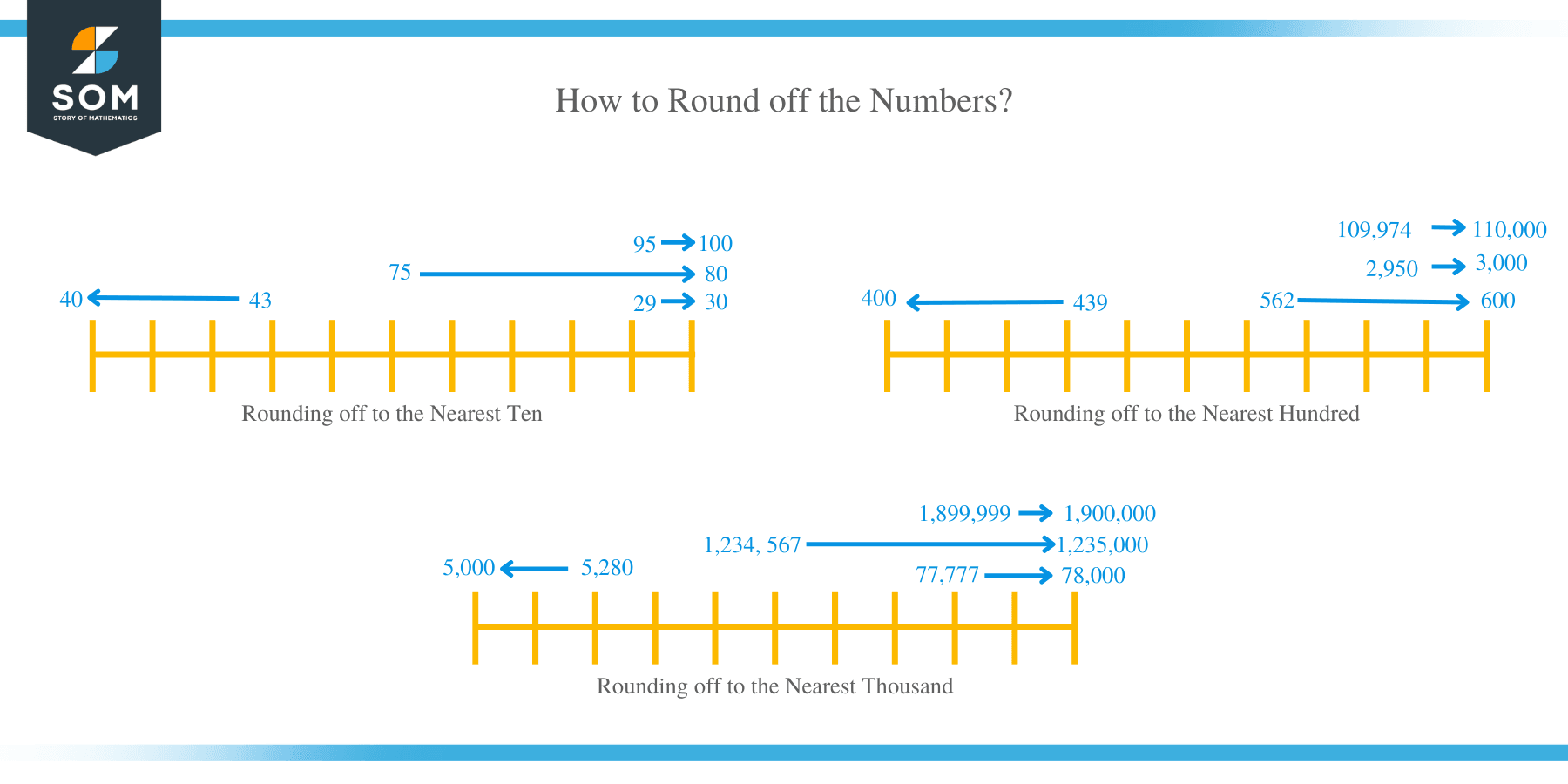# Rounding Numbers – Definition, Place-value Chart & Examples

##What is rounding off numbers?

Rounding off numbers is a mathematical technique of adjusting the number’s digits to make the number easier to use during calculations. Numbers are rounded off to a particular degree of accuracy to make calculations simpler and the results easier to understand.

Before rounding off any number, you should know the place of all digits of a number. Below is the place value chart.

## How to Round off the Whole Numbers?

It is fundamentally important to understand the term “rounding digit” when rounding off numbers.

For example, when rounding off numbers 100 to tens, the rounding digit is the second number from the right. Similarly, the rounding digit is in the third place when rounding to the nearest hundred, which is 1. Therefore, the first step when rounding a number is identifying the rounding digit and looking at the next digit to the right side.

• If the digit to the right side of the rounding digit is 0, 1, 2, 3, or 4, then the rounding digit doesn’t change. All digits to the right of the rounding digit become zero.
• If the digit to the right side of the rounding digit is 5, 6, 7, 8, or 9, the rounding digit increases by one digit. All the digits to the right are dropped to zero.

### Practice questions

1. Round off the following numbers to the nearest tens.

29
95
43
75

2. Round off these numbers to the nearest tens.

164
1,989
765
9,999,995

3. Round the following list of numbers to the nearest hundreds.

439
2,950
109,974
562

4. Round off the numbers below to the nearest thousands.

5,280
1,899,999
77,777
1,234,567

###Solutions

1. Rounding off to the nearest ten:

The digit to the right of the rounding digit in 29 is 9. Therefore, one digit is added to the rounding digit, 2, and the other digit is dropped to zero.

29 → 30

The digit to the right of the rounding digit in 43 is 3. The number does not affect the rounding digit, 4 and 3 are dropped to zero.

43 → 40

The digit to the right of the rounding digit in 75 is 5. One digit is added to the rounding digit, and 5 is dropped to zero.

75 → 80.

The digit to the right of the rounding digit in 95 is 5. One digit is added to 9, and the rest dropped to zero.

95 → 100.

2. Round to the nearest ten:

• 164 has the rounding digit as 6 and 4 as the right-hand side digit.
• 164 will become 160
• 765 will become 770.
• 1,989 → 1,990.
• 9,999,995 has 5 as the digit to the right of the rounding digit.
• 9,999,995 → 10,000,000

3. Rounding off to the nearest hundreds:

Identify the digit to the right (tens digit) of the rounding digit.

The tens digit in 439 is 3, so there is no effect on the hundreds digit:

439 →400

Number 6 is the tens digit or digit to the right of the rounding digit:

562 → 600.

5 is the tens digit in 2,950, so,

2,950 → 3,000.

109,974 has the tens digit as 7.

109,974 then becomes 110,000.

4. To round off to the nearest thousands, the hundreds digit is considered.

The hundred digit in 5,280 is 2, so it does not affect the rounding digit.

5,280, therefore, becomes 5,000.

77,777 becomes 78,000.

1,234, 567 becomes 1,235,000.

1,899,999 becomes 1,900,000.

## How to Round Off Decimal Numbers?

Decimal numbers are rounded off to estimate an answer quickly and easily. Decimal numbers can be rounded off to the nearest integer or whole number, tenths, hundredths, thousandths, etc.

### Rounding off to the nearest integer

The following rules are followed when rounding off a decimal to the nearest whole number:

• The number to be rounded off is identified.
• The digit in one’s place is marked.
• The first digit to the right of the decimal, or in the tenths place, is checked.
• If the tenths digit is less than or equal to 4, then the number in the one’s place is rounded off to a whole number.
• Similarly, if the tenths digit is greater than or equal to 5, add 1 digit to the number in the one’s place.
• Drop all digits after the decimal point to result in the desired whole number.

### Rounding to the nearest tenths

A similar procedure is applied when rounding a number to the nearest tenths.

• The number to be rounded off is first of all identified.
• The digit in the tenths place is marked.
• The digit in the hundredths place is checked.
• If the hundredths digit is less than or equal to 4, then the number in the tenths digit remains the same.
• Similarly, if the hundredths digit is greater than or equal to 5, add 1 digit to the number in the tenths place.
• Drop all digits to the right of the tenths column to result in the desired number.

### Rounding off to the nearest hundredths

The first step is to identify the required number. The digit in the hundredths place is marked. Check whether the digit in the thousandths place is 0, 1, 2, 3, 4 or 5, 6, 7, 8, 9. Round off the number to the nearest hundredths and drop the other numbers to the right of the hundredths place.

### Practice Questions

1. When rounded off to the nearest whole number, $975.6539$ is equal to which number?

2. When rounded off to the nearest whole number, $1240.68$ is equal to which number?

3. When rounded off to the nearest tenths, $845.324$ is equal to which decimal?

4. The length of a road is $27.56$ km. What is the length to the nearest km?

5. The weight of a boy is $37.35$ kg. Calculate the weight to the nearest kg.